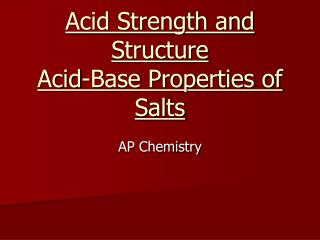DownloadDownload PresentationAcid Strength and Structure Acid-Base Properties of Salts

Acid Strength and Structure Acid-Base Properties of Salts

Télécharger la présentationAcid Strength and Structure Acid-Base Properties of Salts

- - - - - - - - - - - - - - - - - - - - - - - - - - - E N D - - - - - - - - - - - - - - - - - - - - - - - - - - -
Presentation Transcript

1. Structural Considerations of Acids • Acid strength is based on its ability to release a proton (H+) • Any factors that influence this release is important in determining relative acid strength. • Two main factors of strength are: • Strength of the H-X bond • The polarity of the H-X bond

2. Bond Strength HF HCl HBr HI Weakest Acid Strongest Bond Strongest Acid Weakest Bond • HI has a long bond length  strongest acid • HF has a short bond length  weakest acid

3. Bond Polarity and Electronegativity • Increasing electronegativity of associated atoms increase polarity around the proton. • This make it more likely for the proton to dissociate. • Increasing number of oxygen atoms in oxy-acids also increases polarity of the molecule.

4. Strongest Acid HClO4 HClO3 HCLO2 HClO H2SO4 H2SO3 HNO3 HNO2 Weakest acid More oxygens = stronger acid More oxygens means more electrons are pulled away from the proton thereby weakening that bond.

5. Which will be the strongest acid and why? HClO3 HBrO3 HIO3 • Strongest Most electronegative halogen

6. Acid-Base Properties of Salts • Asalt is technically defined as a substance containing a cation other than H+ and an anion other than OH-. • When an acid and base react in a neutralization reaction, a salt and water are always produced. • When a salt dissolves in water it breaks into its ions. • Under certain conditions (depending on the cation and anion in the salt) a salt solution can be acidic or basic.

7. 1.) The salt produced by the reaction of a strong acid and a strong base is always neutral. (NaCl, KCl, NaNO3) NaCl  Na+ + Cl- • The cation comes from the base • The anion comes from the acid • Cl- is the conjugate base of a strong acid HCl. It wants to stay as an ion! • Na+ is the conjugate acid of a strong base NaOH. It also wants to stay an ion • It will not attack water (hydrolysis) and change the pH

8. 2) The salt produced by the reaction of a weak acid and a strong base is basic. (NaC2H3O2, NaF) • F- is the conjugate base of a weak acid HF • This makes F- as strong base! • It will attack water (hydrolyze) as follows: F- + H2O  HF + OH- • This increases [OH-] making the solution basic!

9. 3.) The salt produced by the reaction of a strong acid and a weak base is acidic. (NH4Cl, NH4NO3) • NH4+ is the conjugate acid of a weak base, NH3 • This makes NH4+ a strong acid. • It will give water its H+ ion in solution as follows: NH4+ + H2O  H3O+ + NH3 • This increases [H+] making the solution acidic!

10. 4.) A salt made up of a highly charge metal ion and a strong acid will be acidic. (FeCl3, Al(NO3)3) • When the Fe3+ is solvated by water the high charge pulls electrons away from oxygen, which then in turn pulls electrons away from the hydrogens with more force. • This weakens the H-O bond and allows the H+ to dissociate more easily. H Fe3+ ---- O H

11. 5.)The salt produced by the reaction of a weak acid and a weak base depends of the Ka of the acid: (NH4CN, NH4C2H3O2) • If Ka>Kb, an acidic salt results. • If Ka<Kb, a basic salt results. • If Ka = Kb, a neutral salt results.

12. An important relationship at 25oC: Ka x Kb = Kw • Where Ka is the dissociation constant for the acid and Kb is the dissociation constant for the conjugate base.

13. Determining pH of Salt Solutions • What is the pH of a 0.5M NaF solution. The Ka of HF is 7.2 x 10-4. • In an aqueous solution, NaF will completely dissociate (its an ionic compound) and the following equilibrium exists: • F-(aq)+ H2O(l) ⇄ HF(aq)+OH-(aq) • The Na+ has no acid or base properties and therefore is not considered in the equilibrium, Since HF is a weak acid, its conjugate base, F- should be considered a strong base. Therefore the Kb expression will be important: • Ka x Kb = Kw so: • Kb = Kw/Ka = 1.39x10-11

14. F- (aq)+ H2O(l) ⇄ HF(aq) + OH-(aq) Initial: 0.5M ----- 0 0 Equil. : 0.5 – x ----- x x • 1.39 x 10-11 = x2 or 1.39 x 10-11 = x2 0.5 – x 0.5 • x = 5.3 x 10-6 M • x = [OH-], so pOH = 5.28 and pH = 8.72 • As expected, the solution is basic.